## 2、阿拉伯数字对应大写表 - 数字转大写金额 (以下红色字可选中复制)

•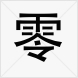0 =
•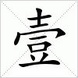1 =
•2 =
•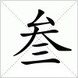3 =
•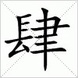4 =
•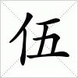5 =
•6 =
•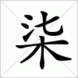7 =
•8 =
•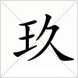9 =

## 3、金额单位(位数)对应表

•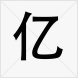亿 = 亿
•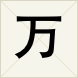万 =
•千 =
•百 =
•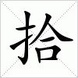十 =
•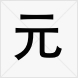元 = 元(圆)
•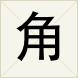角 =
•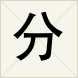分 =
•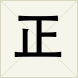正 =
•整 =